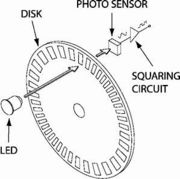# synchronizing the speed of two motors using rotary encoders

hey pals, i am trying to synchronize the speed of two motors using rotary encoders (which look like the one in the attached image). my basic is approach is to count the number of pulses that is generated by each encoder and than compare them with each other. now the encoder generating the greater number of 1's shows that the speed of that motor is greater than the other so it's speed is reduced by sending a lower pwm as input.

now this approach is very vague, i am new to arduino and don't really know how to use interrupts and timers. a bit of help will be appreciated ...
here is the coding i have done so far.
note:
i'm giving input to the motors through h-bridges.

``````int enc1=22; //1st encoder
int enc2=24; //2nd encoder
int count1=0; //counter for number of 1's generated by the encoder
int count2=0;
// h-bridge pins for motor 1
int a=9;
int b=10;
int c=11;
int d=12;
//pins for motor 2
int a1=2;
int b1=3;
int c1=7;
int d1=8;

int p=220; //initial pwn for motor 1
int p1=220; //initial pwn for 2ns motor

void setup()
{
Serial.begin(9600);
}

void loop()
{
int i=360;
if (i>0)
{
forward(p,p1);

if(a==1) {count1=count1+1;}
if(b==1) {count2=count2+1;}

Serial.println("count1:");
Serial.println(count1);
Serial.println("count2:");
Serial.println(count2);
i--;
}
if (count1>count2)
{p=200;}
else if (count2>count1)
{p1=200;}

}

void forward(int p, int p1)
{
forw(p);
forw1(p1);
}
void stopp(int p, int p1)
{
st(p);
st1(p1);
}
void backwards(int p, int p1)
{
back(p);
back1(p1);
}
void left(int p, int p1)
{
st(p);
forw1(p1);
}
void right(int p, int p1)
{
forw(p);
st1(p1);
}

void forw(int p)
{
digitalWrite(a,p); // high, pwm of 94% dutycycle
digitalWrite(b,LOW); // low
digitalWrite(c,LOW);
digitalWrite(d,p);
}

void forw1(int p)
{
digitalWrite(a1,p); // high, pwm of 94% dutycycle
digitalWrite(b1,LOW); // low
digitalWrite(c1,LOW);
digitalWrite(d1,p);
}

void st(int p)
{
digitalWrite(a,LOW);
digitalWrite(b,LOW);
digitalWrite(c,p);
digitalWrite(d,p);
}
void st1(int p)
{
digitalWrite(a1,LOW);
digitalWrite(b1,LOW);
digitalWrite(c1,p);
digitalWrite(d1,p);
}

void back(int p)
{
digitalWrite(a,LOW);
digitalWrite(b,p);
digitalWrite(c,p);
digitalWrite(d,LOW);
}
void back1(int p)
{
digitalWrite(a1,LOW);
digitalWrite(b1,p);
digitalWrite(c1,p);
digitalWrite(d1,LOW);
}
``````Please read the how to use this forum sticky and post you code correctly. Basically this is a servo problem with feedback, there is a limit yio how far you can drag the motor speed with PWM.
If it were me I would a phase locked loop to synchronise the motors and leave the arduino out altogether.

but i am required to use arduino for the purpose. please give some ideas about hoe can i use the feedback thing through arduino...
i just need to maintain the speed of both motors to a moderate level, so that my robotic platform should not drift from the straight path...

Robots, why keep that a secret until now?
And you are required, so that means it is an assignment.

Your code has to measure the two counts and adjust the PWM level if the two are not equal. This means putting the speed in a varialbe and incrementing or decrementing that variable depending on the counts. Arrange each to trigger an interrupt to increment the count. Keep one motor a constant speed and just change the other. When the counter gets too big, approaching the limit of an int variable then subtract the same large number from each count.

Your code has to measure the two counts and adjust the PWM level if the two are not equal. This means putting the speed in a varialbe and incrementing or decrementing that variable depending on the counts

hey that's helpful, and it's all i'm stuck at...is it the right way that i used in my program for the count of encoder's readings? can you please help me out in how to form some sort of formula to calculate the speed and changing it according to the encoder value?

is it the right way that i used in my program for the count of encoder's readings?

no, look at using interrupts to do the counting.
Start here
http://playground.arduino.cc/Code/Interrupts
You need two ISRs one for each counter, all they do is increment a counter, be sure to declare the count variables as volatile inc.

can you please help me out in how to form some sort of formula to calculate the speed and changing it according to the encoder value?

No that is not how it works, there are too many variables that you will never be able to find out. It is a trial and error algorithm. As long as you decrement the speed control when it needs to go slower it will eventually lock into the right speed. There are maths involved in this but they are first, possibly second year undergraduate level.

thanks a lot! i'm working with interrupts now, you and that link are awesomely helpful